# Hierarchical Clustering 階層式分群 | Clustering 資料分群 | R 統計

Hierarchical Clustering, 屬於資料分群的一種方法。資料分群屬於非監督式學習，處理的資料是沒有正確答案/標籤/目標變數可參考的。常見的分群方法包括著名的kmeans, hierarchical clustering，分別使用不同分群演算邏輯。本篇將介紹階層式分群的實作與特色。

### 資料分群簡介

• 資料分群是一個將資料分割成數個子集合的方法，主要目的包括：
• 找出資料中相近的群聚(clusters)
• 找出各群的代表點。代表點可以是群聚中的中心點(centroids)或是原型(prototypes)
• 透過各群的代表點，可以達到幾個目標：
• 資料壓縮
• 降低雜訊
• 降低計算量
•  資料分群將依據資料自身屬性計算彼此間的相似度（物以類聚），而「相似度」主要有兩種型態：
• 「Compactness」：目標是讓子集合間差異最大化，子集合內差異最小化。如階層式分群和K-means分群。
• 「Connectedness」：目標是將可串連在一起的個體分成一群。如譜分群(Spectral Clustering)。
• 資料分群屬於非監督式學習法(Unsupervised Learning)，即資料沒有標籤(unlabeled data)或沒有標準答案，無法透過所謂的目標變數(response variable)來做分類之訓練。也因為資料沒有標籤之緣故，與監督式學習法和強化式學習法不同，非監督式學習法無法衡量演算法的正確率

1. 階層式分群(hierarchical clustering)
1. 聚合式階層分群法 Agglomerative Hierarchical Clustering
2. 分裂式階層分群法 Divisive Hierarchical Clustering
3. 最佳分群群數(Determining Optimal Clusters)
2. 切割式分群(partitional clustering)
1. K-means
2. K-medoid
3. 最佳分群群數(Determining Optimal Clusters)
3. 譜分群(Spectral Clustering)

### 1. 階層式分群(Hierarchical clustering)

• 階層分群法的概念是在分群中建立分群，並不需要預先設定分群數。
• 產生的分群結果為一目瞭然的樹狀結構圖（又稱作dendrogram）。
• 群數(number of clusters)可由大變小(divisive hierarchical clustering)，或是由小變大(agglomerative hierarchical clustering)，透過群聚反覆的分裂和合併後，在選取最佳的群聚數。
• 階層式分群兩種演算法：
• 當採行聚合法，又稱作AGNES(Agglomerative Nesting)，資料會由樹狀結構的底部開始開始逐次合併 (bottom-up)，在R語言中所使用的函數為hclust()[in stat package]或agnes[in cluster package]擅於處理與識別小規模群聚
• 當採行分裂法，又稱作DIANA(Divisive Analysis)，資料則會由樹狀結構的頂部開始逐次分裂(top-down)，在R語言中使用的套件為diana()[in cluster package]擅於處理與識別大規模群聚
• 階層分群可被用運用數值與類別資料。

### 1-1. 聚合式階層群聚法（AGNES, bottom-up）

• d : 由dist()函數計算出來資料兩兩間的相異度矩陣(dissimilarity matrix)，即兩兩資料間的距離矩陣。
• method: 群(clusters)聚合連結的方式。包括：single(單一）、complete（完整）、average（平均）、Ward’s（華德）和 centroid（中心）等法。其中又以average(平均)聚合方法被認為是最適合的。不同方法對階層分群結果亦有極大影響。

R語言hclust()套件中提供群聚距離演算法衡量兩群聚的不相似度，最常見的幾種演算法如下：

$$d(C_{i},C_{j})=\min_{a\in C_{i},b\in C_{j}}d(a,b)$$
$$d(C_{i},C_{j})=\max_{a\in C_{i},b\in C_{j}}d(a,b)$$
$$d(C_{i},C_{j})=\sum_{a\in C_{i},b\in C_{j}}\frac{d(a,b)}{|C_{i}||C_{j}|}$$
其中，$$|C_{i}|$$和$$|C_{j}|$$分別為群聚$$C_{i}$$和$$C_{j}$$的大小。
$$d(A,B)= d(\bar{X}_{A},\bar{X}_{B})=\|\bar{X}_{A}-\bar{X}_{B}\|^2$$
• 華德最小變異法(Ward’s Minimum Variance): 最小化各群聚內變異加總(minimize the total within-cluster variance)。主要用來尋找緊湊球型的群聚。反覆比較每對資料合併後的群內總變異數的增量，並找增量最小的組別優先合併。越早合併的子集表示其間的相似度越高。而使用華德最小變異法的前提為，初始各點資料距離必須是歐式距離的平方和(Squared Euclidean Distance)。在R套件hclust將參數設為method = “ward.D2″(不是”ward.D”)。（華德法參考文獻

• 歐式距離（以二維空間為例）：最常用也是最重要的距離計算法
$$d(P_{1},P_{2})=\sqrt{(x_{1}-x_{2})^2+(y_{1}-y_{2})^2}$$
• 曼哈頓距離（以二維空間為例）：
$$d(P_{1},P_{2})=|x_{1}-x_{2}|+|y_{1}-y_{2}|$$

• Height表示n-1組實數值。各值為使用特定聚合方法計算出來的標準數值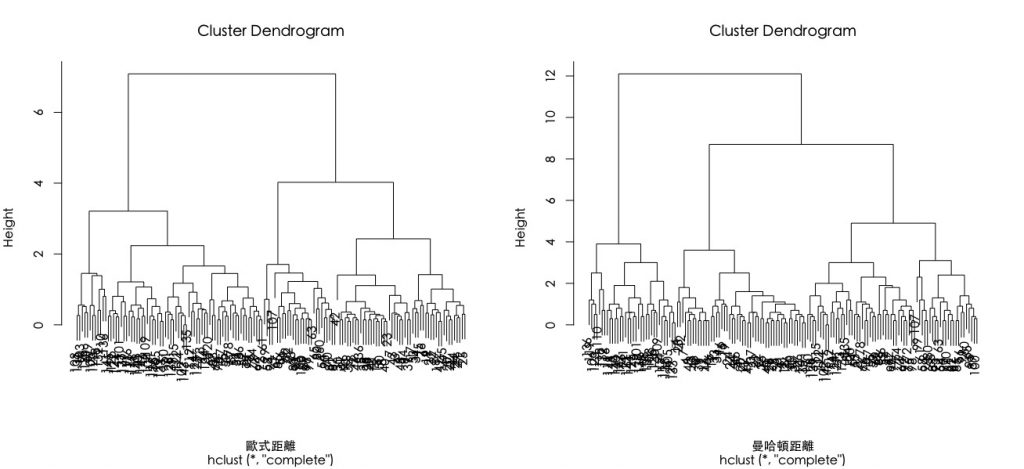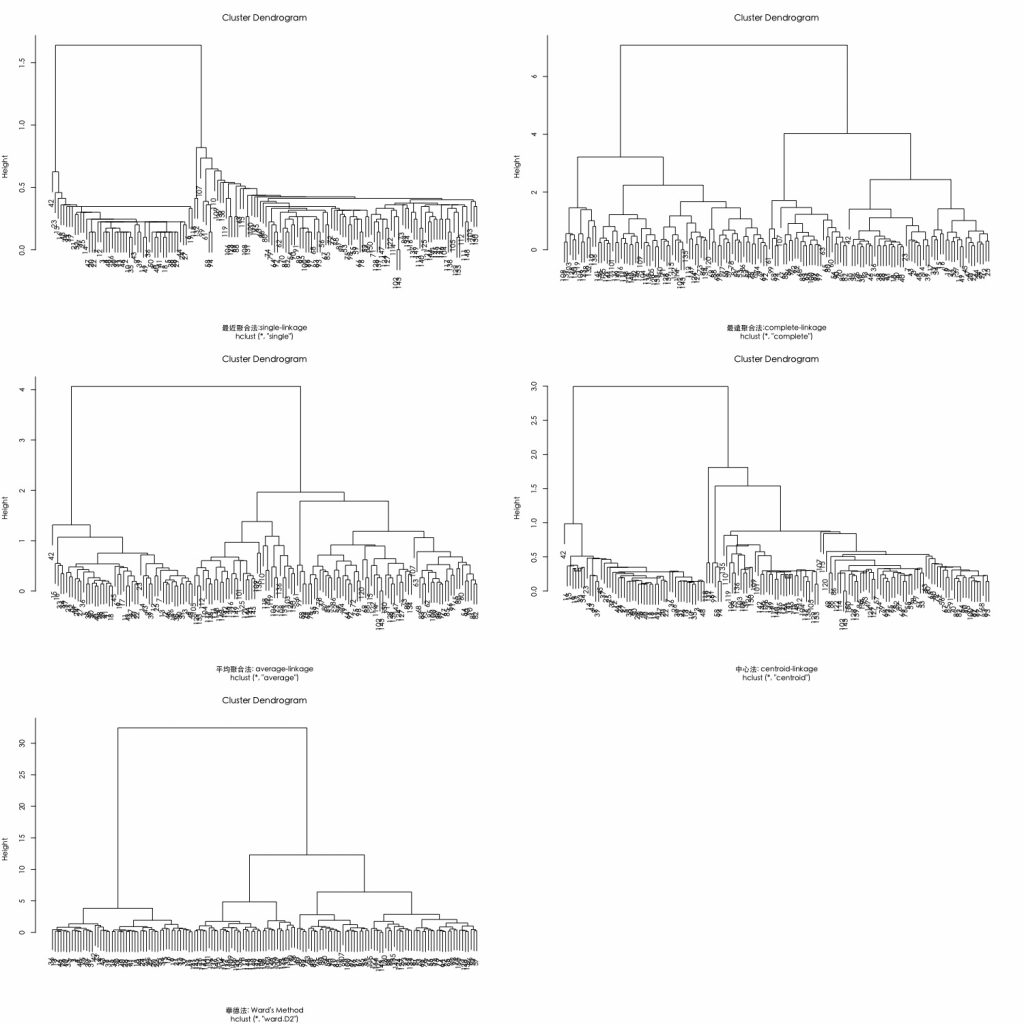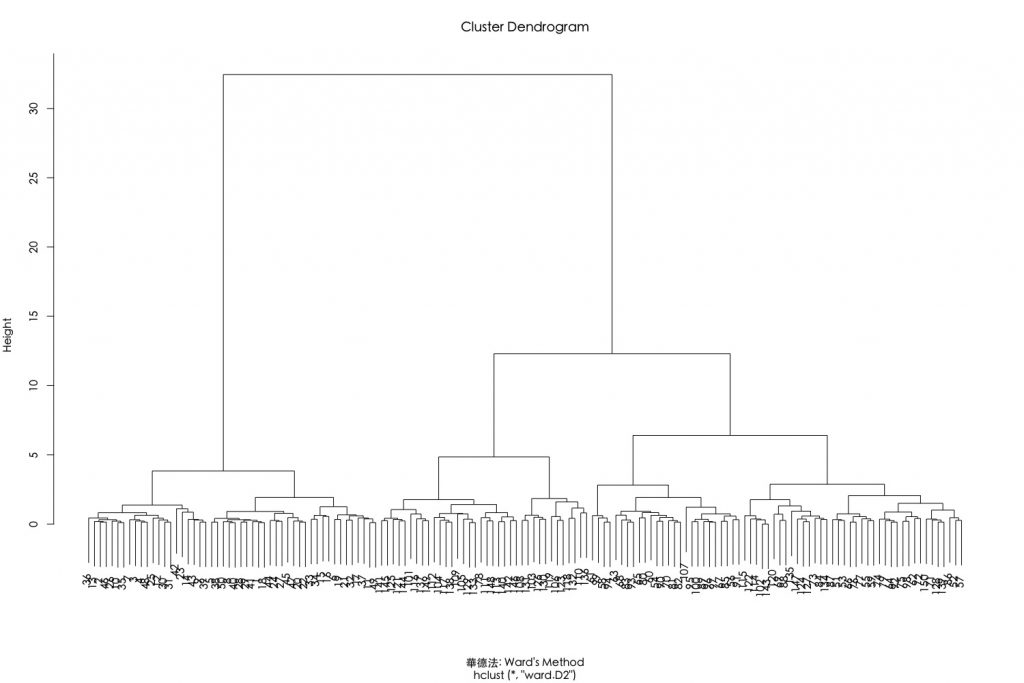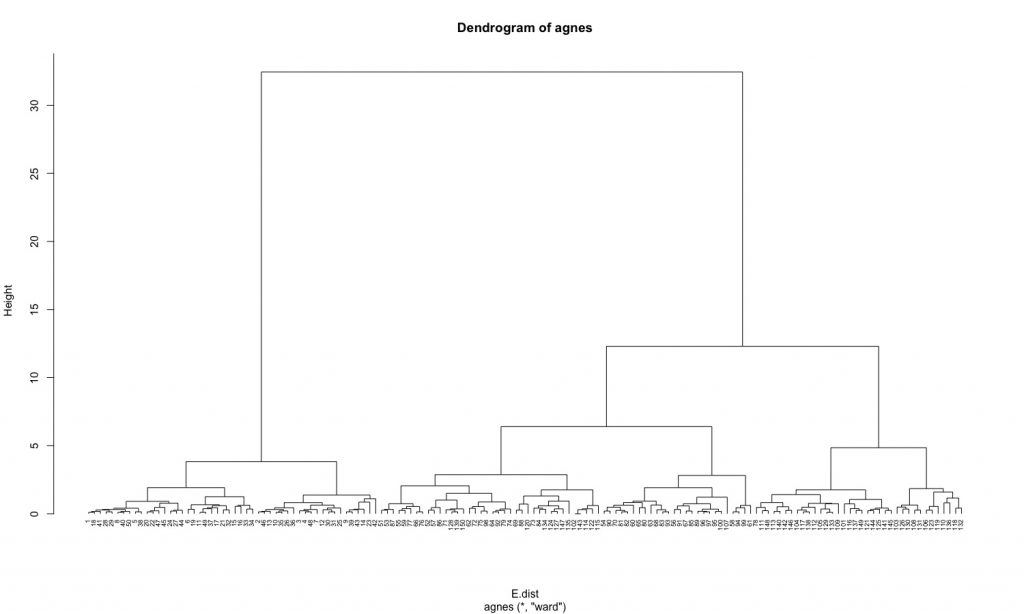1. 指定所要的分群數: rect.hclust(k=…)、cutree(k=…)
2. 指定截枝的位置: rect.hclust(h=…)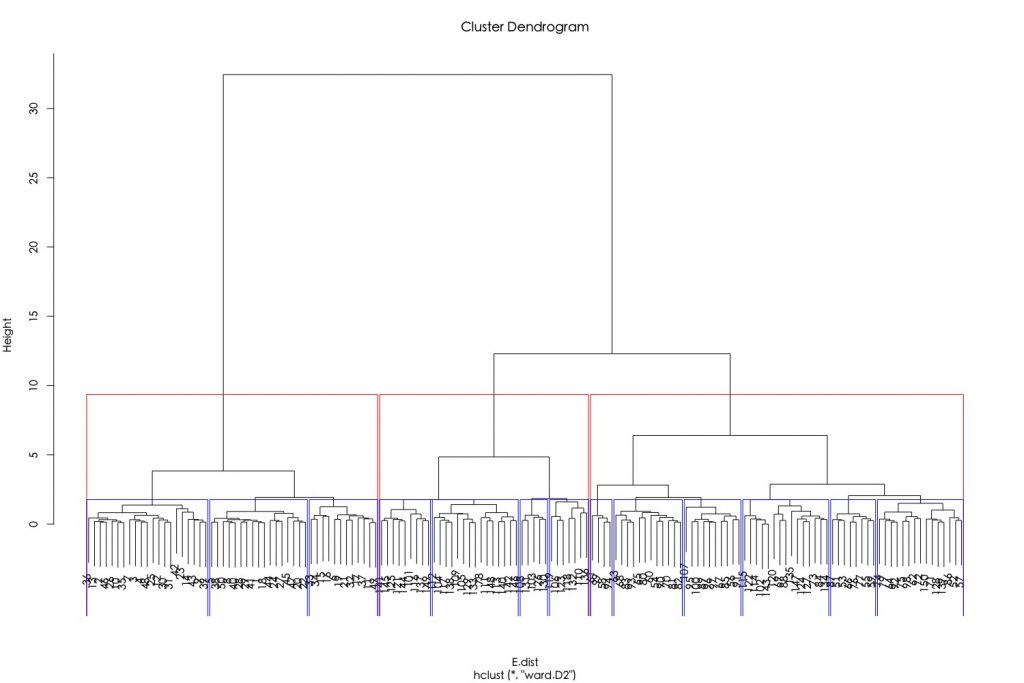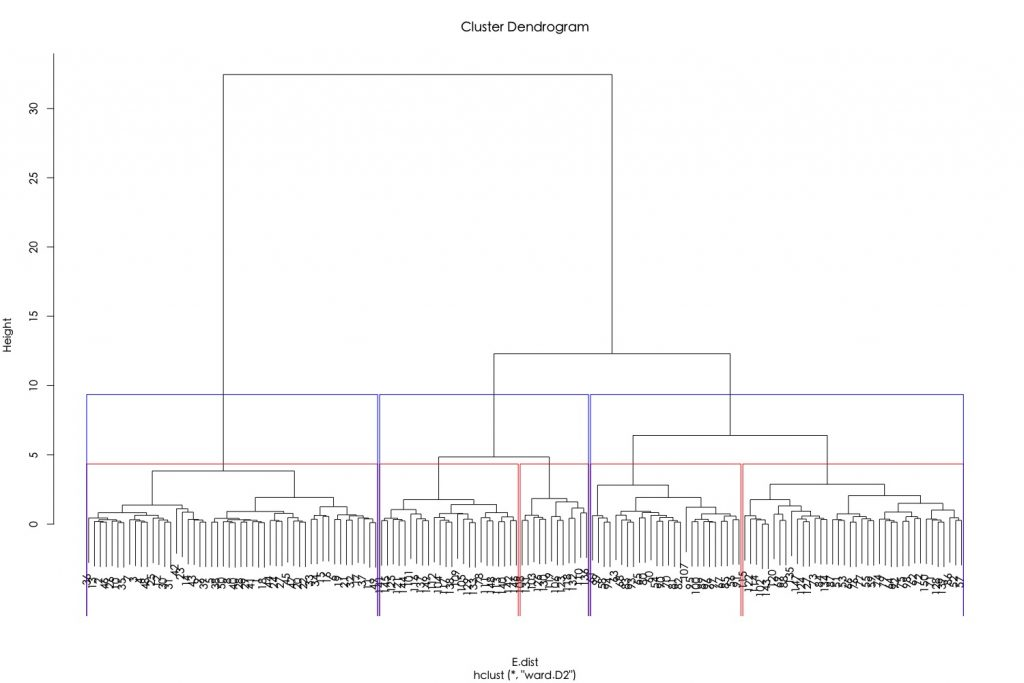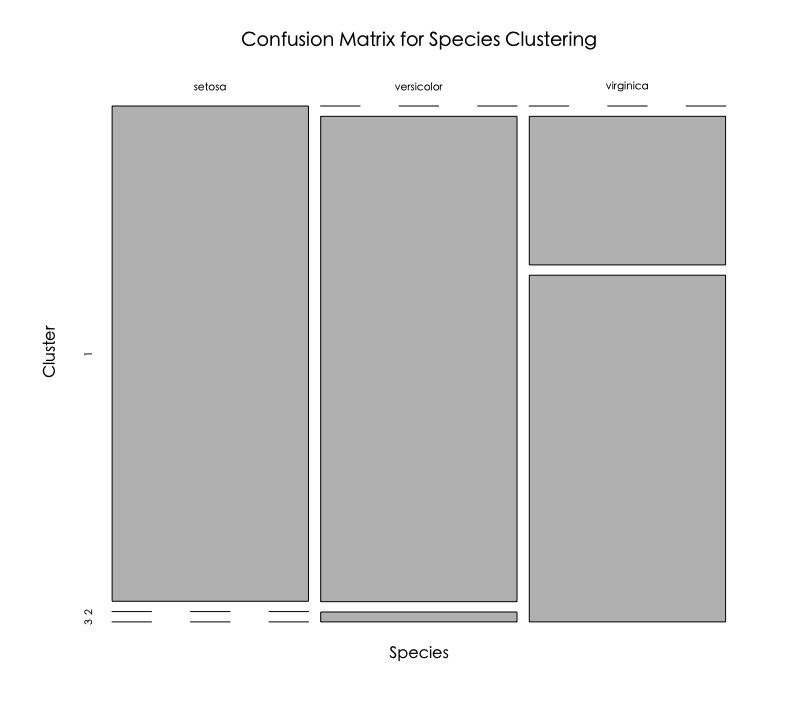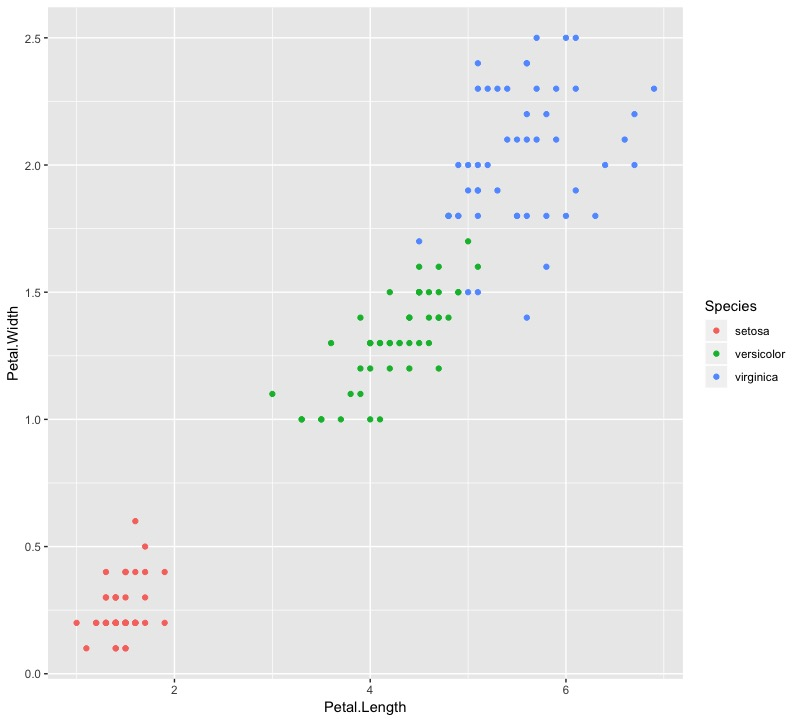1-2. 分裂式階層群聚法（DIANA, top-down）

diana()函數運作方式很類似agnes()，只差diana沒有method參數

• 葉節點（末梢節點）代表個別資料點。
• 垂直座標軸的Height代表群聚間的(不)相似度((dis)similarity)， 群聚的高度(height)越高，代表觀測值間越不相似（組內變異越大）。需要注意的是，要總結兩個觀測值的相似度，只能用兩觀測值何時被第一次合併的群聚高度來做判斷，而不能以水平軸的距離來評估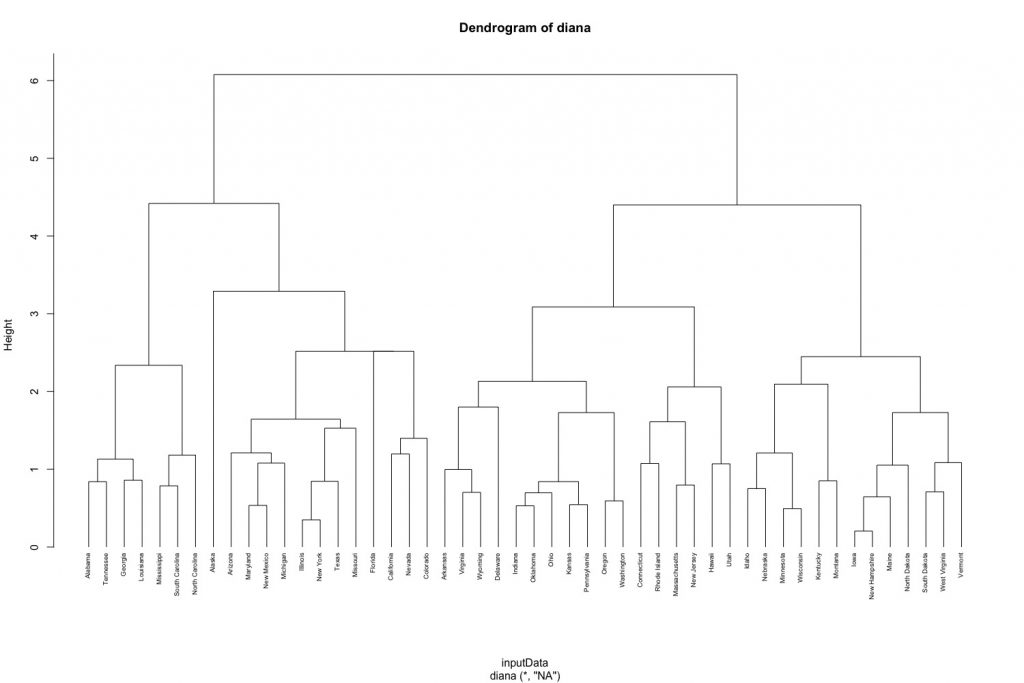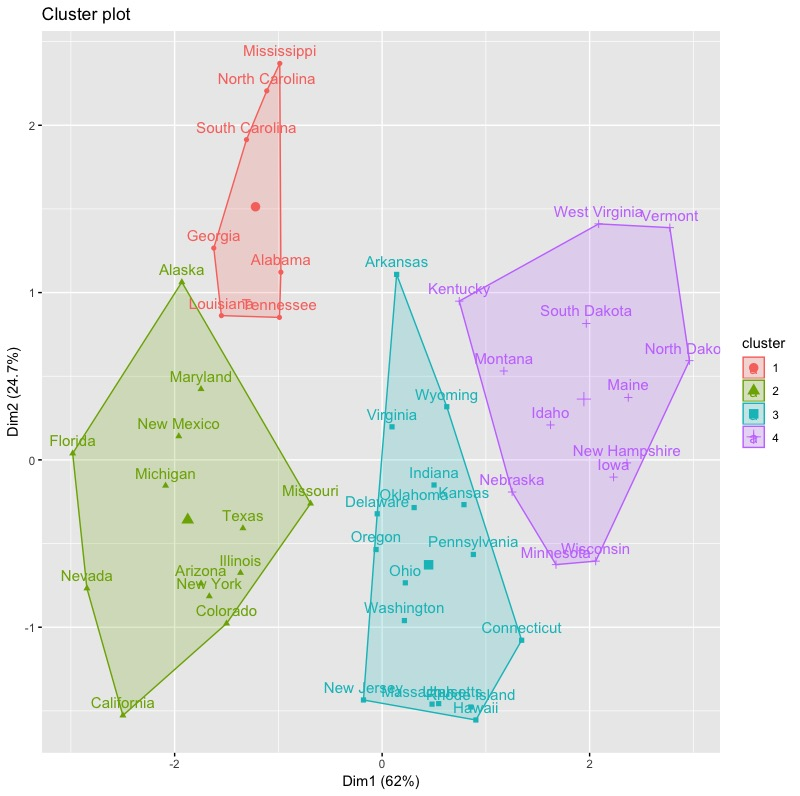### 1-3. 決定階層式分群最佳群聚數

#### 1-3-1. Elbow Method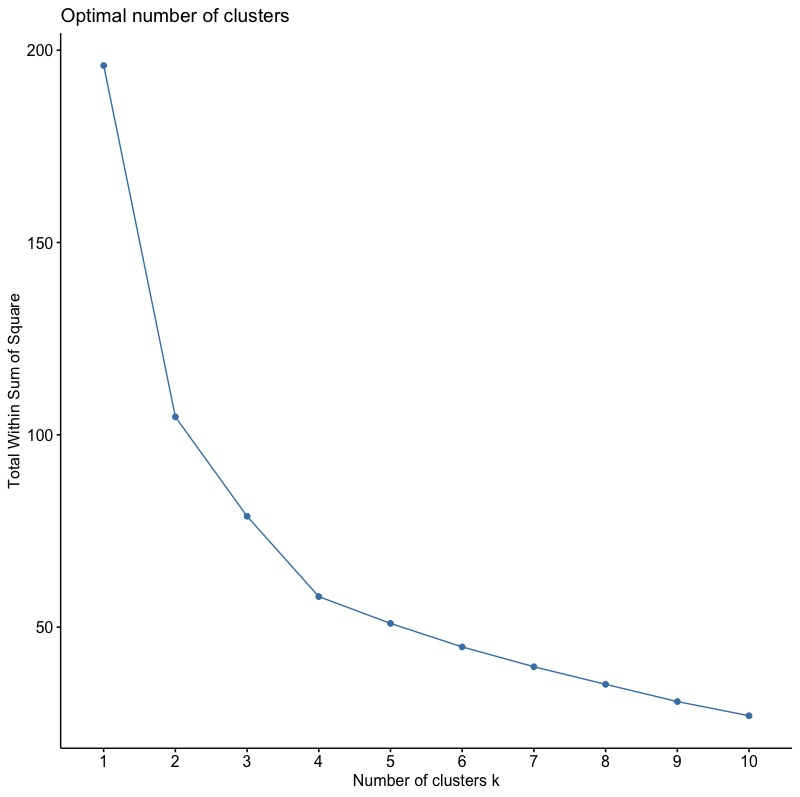#### 1-3-2. Average Silhouette Method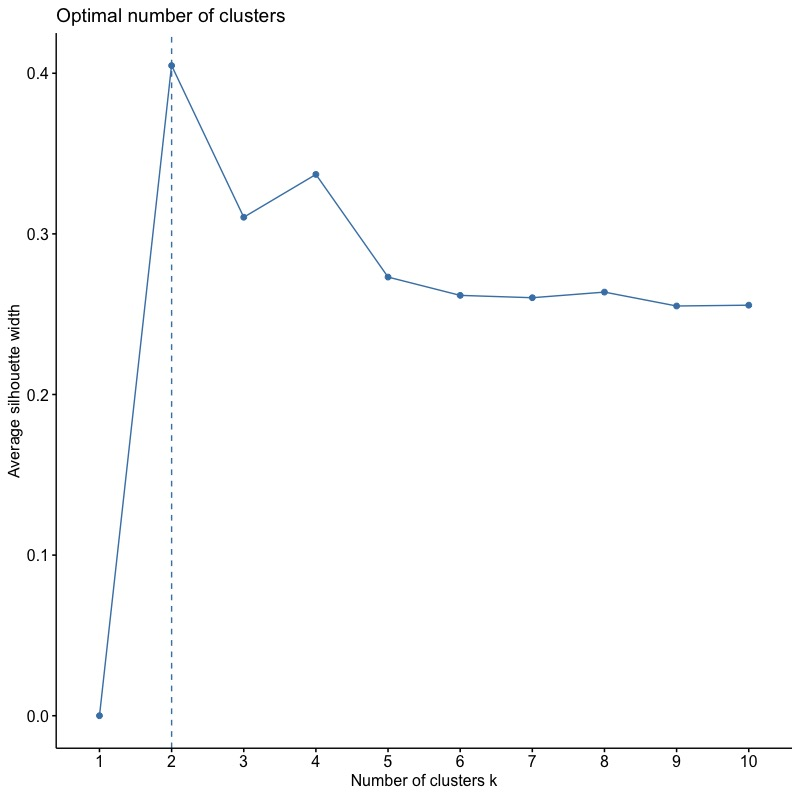#### 1-3-3. Gap Statistic Method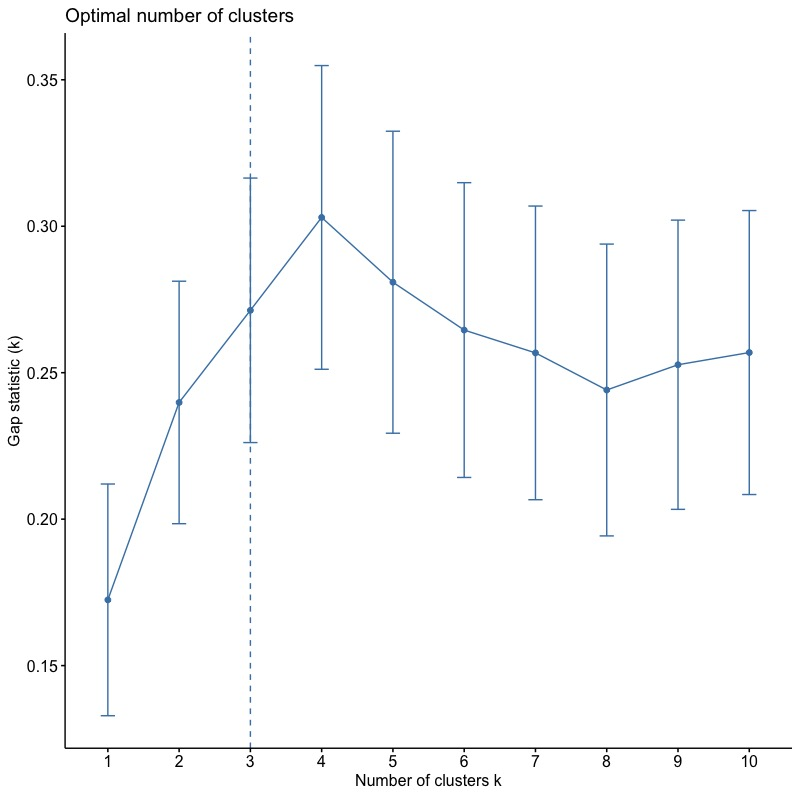### 總結

• 階層分群主要受：資料個體兩兩間距離矩陣衡量方法群與群連結方法所影響
•  階層分群優點包括：
• 概念簡單且樹狀圖(dendrogram)結果一目瞭然。
• 只需要資料點間距離即可產出分群結果。
• 可以應用在數值或類別資料。
• 但缺點就是運算速度，以及不適用處理大量資料。運算速度方面，可以考慮其他R套件如fastcluster中的hclust函數，執行上會比一般hclust更有效率。

#### 在《Hierarchical Clustering 階層式分群 | Clustering 資料分群 | R 統計》中有 6 則留言

1.Amy表示:

請問文中提及階層分群可被用運用數值與類別資料，但如果是類別資料，我應該要如何定義變項的數值及如何計算其距離??

1.jamleecute表示:

如遇類別型資料，在計算dissimilarity matrix 使用的距離計算方式是Gower distance(而非傳統的Eucledian distances)，可以使用cluster package中的daisy()函數處理，可以處理混合形態的變數。
R函數計算方式為 gower.dist https://www.rdocumentation.org/packages/cluster/versions/2.0.6/topics/daisy
希望有回答到你的問題^^

2.Li表示:

最近才發現大大的網站，相見恨晚><
超級厲害！受用無窮呀！
非常感謝Orz

3.Alex表示:

打擾囉!針對您所提供的daisy()函數，來處理資料中有連續型態，和類別型態變數使用，
想請問，這樣我資料需要預處理，使其標準化後，再套用該函數嗎?
亦或直接將所分析之資料變數放進去即可呢?

4.Alex表示:

放進去即可呢?打擾囉!針對您所提供的daisy()函數，來處理資料中有連續型態，和類別型態變數使用，
想請問，這樣我資料需要預處理，使其標準化後，再套用該函數嗎?
亦或直接將所分析之資料變數